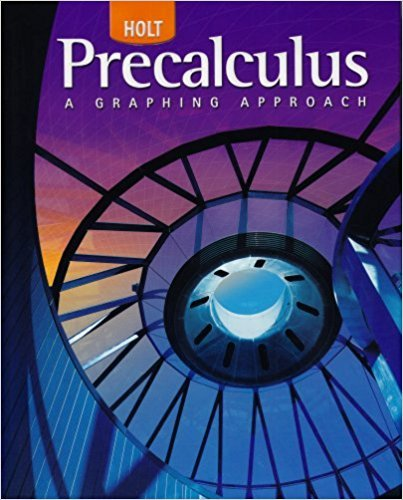×
×

# Solutions for Chapter 14.5: Limits Involving Infinity## Full solutions for Precalculus | 1st Edition

ISBN: 9780030416477Solutions for Chapter 14.5: Limits Involving Infinity

Solutions for Chapter 14.5
4 5 0 306 Reviews
10
1
##### ISBN: 9780030416477

Since 50 problems in chapter 14.5: Limits Involving Infinity have been answered, more than 24481 students have viewed full step-by-step solutions from this chapter. This expansive textbook survival guide covers the following chapters and their solutions. Chapter 14.5: Limits Involving Infinity includes 50 full step-by-step solutions. This textbook survival guide was created for the textbook: Precalculus, edition: 1. Precalculus was written by and is associated to the ISBN: 9780030416477.

Key Calculus Terms and definitions covered in this textbook
• Back-to-back stemplot

A stemplot with leaves on either side used to compare two distributions.

• Direct variation

See Power function.

• Directed line segment

See Arrow.

• Division algorithm for polynomials

Given ƒ(x), d(x) ? 0 there are unique polynomials q1x (quotient) and r1x(remainder) ƒ1x2 = d1x2q1x2 + r1x2 with with either r1x2 = 0 or degree of r(x) 6 degree of d1x2

• Exponential decay function

Decay modeled by ƒ(x) = a ? bx, a > 0 with 0 < b < 1.

• First-degree equation in x , y, and z

An equation that can be written in the form.

• Fitting a line or curve to data

Finding a line or curve that comes close to passing through all the points in a scatter plot.

• Imaginary unit

The complex number.

• Implied domain

The domain of a function’s algebraic expression.

• Intermediate Value Theorem

If ƒ is a polynomial function and a < b , then ƒ assumes every value between ƒ(a) and ƒ(b).

• Interval

Connected subset of the real number line with at least two points, p. 4.

• Line of symmetry

A line over which a graph is the mirror image of itself

• n-set

A set of n objects.

• Removable discontinuity at x = a

lim x:a- ƒ(x) = limx:a+ ƒ(x) but either the common limit is not equal ƒ(a) to ƒ(a) or is not defined

• Residual

The difference y1 - (ax 1 + b), where (x1, y1)is a point in a scatter plot and y = ax + b is a line that fits the set of data.

• Scatter plot

A plot of all the ordered pairs of a two-variable data set on a coordinate plane.

• Semimajor axis

The distance from the center to a vertex of an ellipse.

• Sine

The function y = sin x.

• Terminal side of an angle

See Angle.

• Vertices of an ellipse

The points where the ellipse intersects its focal axis.

×